Скачать презентацию Money and Inflation An introduction Introduction

bdb055cbc4f3f6e83910b2f481e7cda0.ppt

• Количество слайдов: 17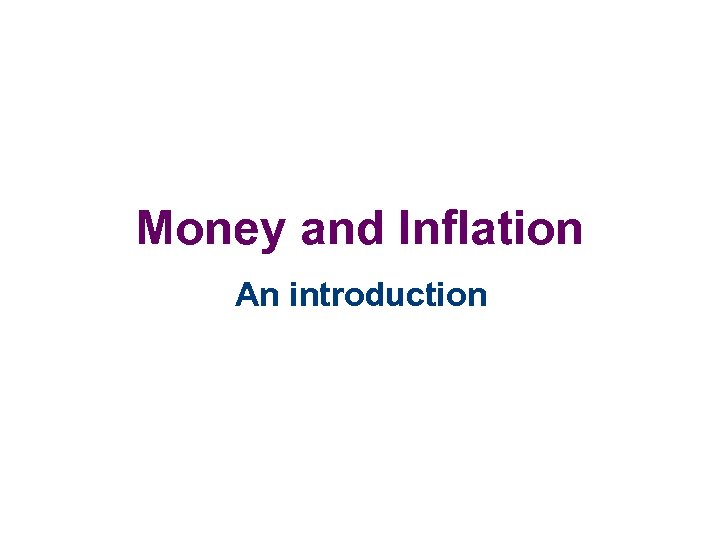Money and Inflation An introduction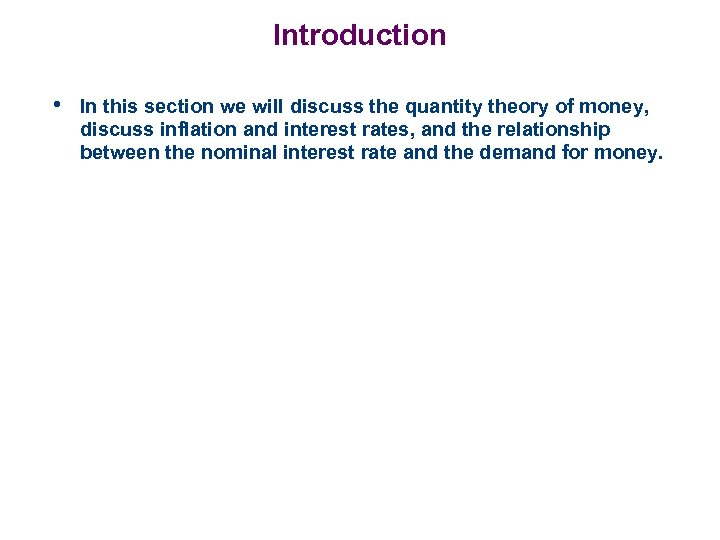Introduction • In this section we will discuss the quantity theory of money, discuss inflation and interest rates, and the relationship between the nominal interest rate and the demand for money.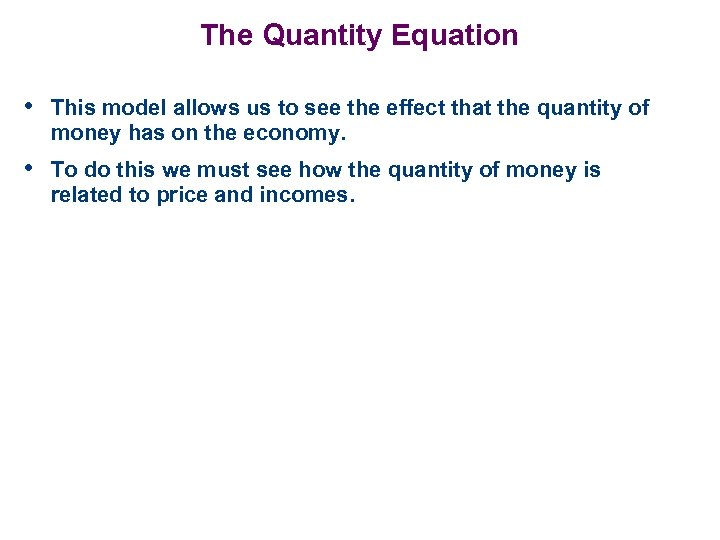The Quantity Equation • This model allows us to see the effect that the quantity of money has on the economy. • To do this we must see how the quantity of money is related to price and incomes.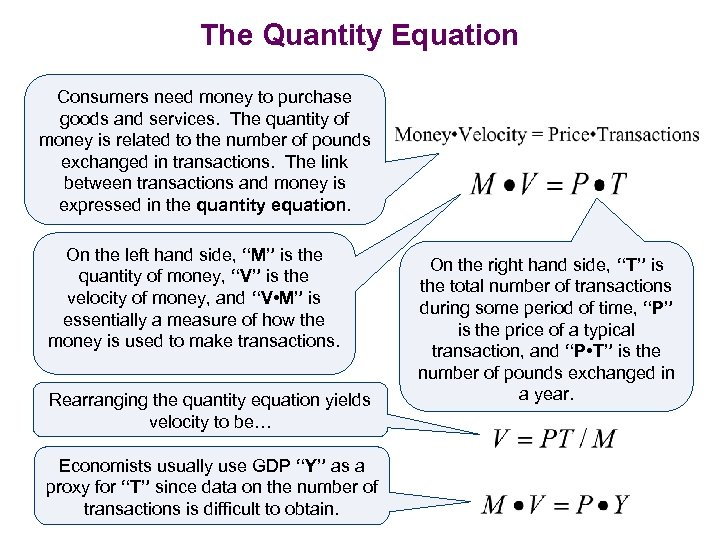The Quantity Equation Consumers need money to purchase goods and services. The quantity of money is related to the number of pounds exchanged in transactions. The link between transactions and money is expressed in the quantity equation. On the left hand side, “M” is the quantity of money, “V” is the velocity of money, and “V • M” is essentially a measure of how the money is used to make transactions. Rearranging the quantity equation yields velocity to be… Economists usually use GDP “Y” as a proxy for “T” since data on the number of transactions is difficult to obtain. On the right hand side, “T” is the total number of transactions during some period of time, “P” is the price of a typical transaction, and “P • T” is the number of pounds exchanged in a year.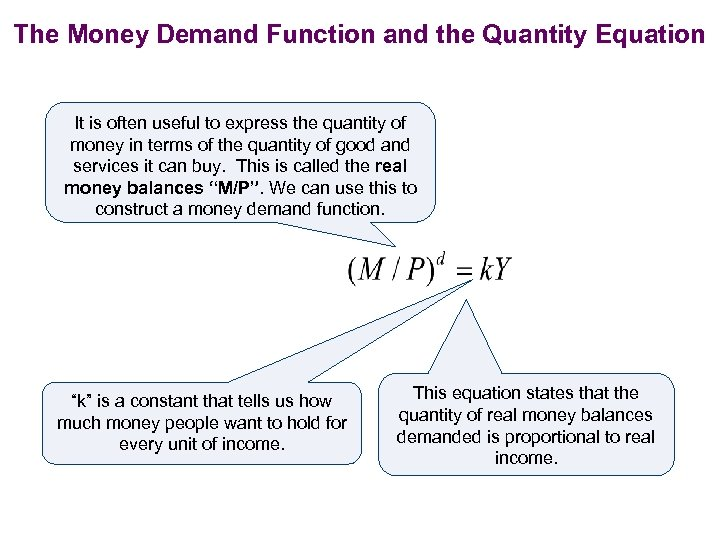The Money Demand Function and the Quantity Equation It is often useful to express the quantity of money in terms of the quantity of good and services it can buy. This is called the real money balances “M/P”. We can use this to construct a money demand function. “k” is a constant that tells us how much money people want to hold for every unit of income. This equation states that the quantity of real money balances demanded is proportional to real income.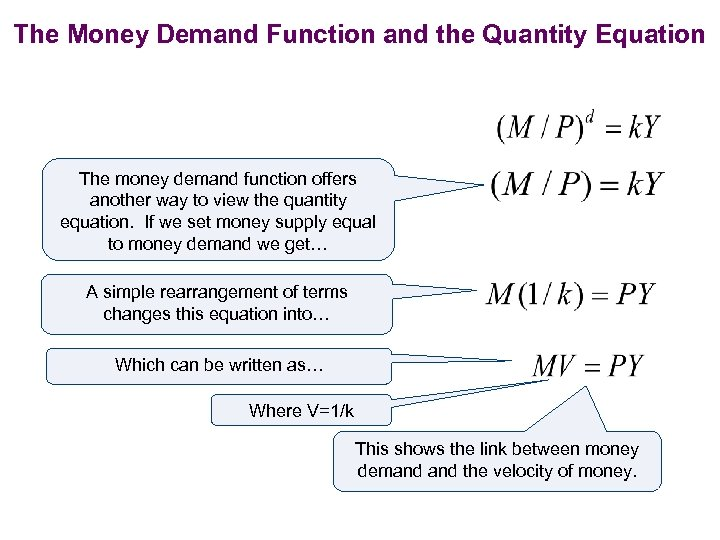The Money Demand Function and the Quantity Equation The money demand function offers another way to view the quantity equation. If we set money supply equal to money demand we get… A simple rearrangement of terms changes this equation into… Which can be written as… Where V=1/k This shows the link between money demand the velocity of money.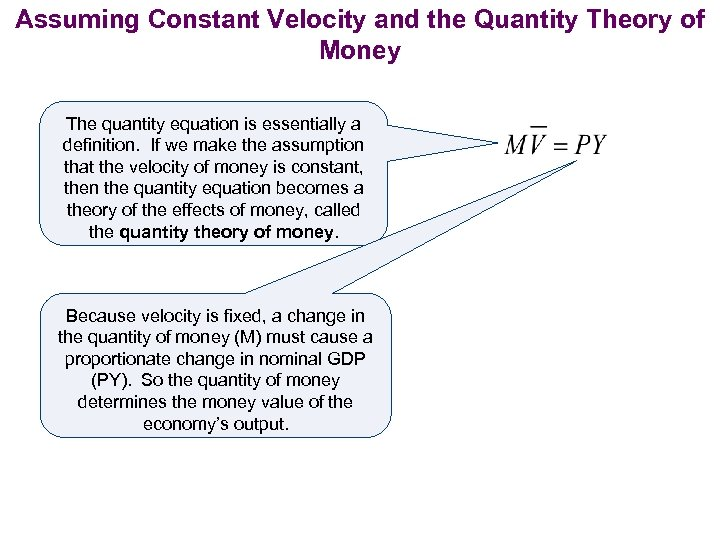Assuming Constant Velocity and the Quantity Theory of Money The quantity equation is essentially a definition. If we make the assumption that the velocity of money is constant, then the quantity equation becomes a theory of the effects of money, called the quantity theory of money. Because velocity is fixed, a change in the quantity of money (M) must cause a proportionate change in nominal GDP (PY). So the quantity of money determines the money value of the economy’s output.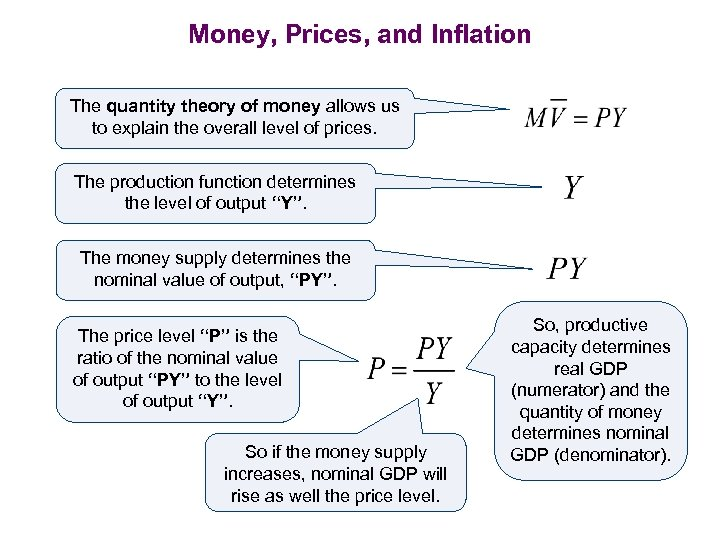Money, Prices, and Inflation The quantity theory of money allows us to explain the overall level of prices. The production function determines the level of output “Y”. The money supply determines the nominal value of output, “PY”. The price level “P” is the ratio of the nominal value of output “PY” to the level of output “Y”. So if the money supply increases, nominal GDP will rise as well the price level. So, productive capacity determines real GDP (numerator) and the quantity of money determines nominal GDP (denominator).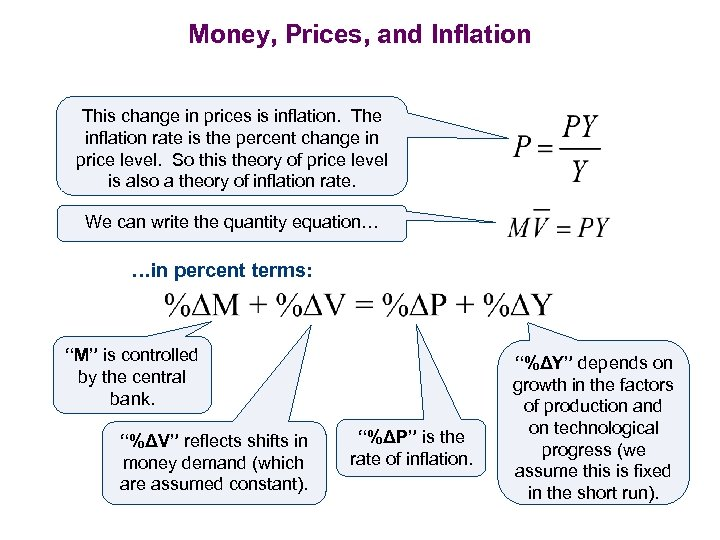Money, Prices, and Inflation This change in prices is inflation. The inflation rate is the percent change in price level. So this theory of price level is also a theory of inflation rate. We can write the quantity equation… …in percent terms: “M” is controlled by the central bank. “%ΔV” reflects shifts in money demand (which are assumed constant). “%ΔP” is the rate of inflation. “%ΔY” depends on growth in the factors of production and on technological progress (we assume this is fixed in the short run).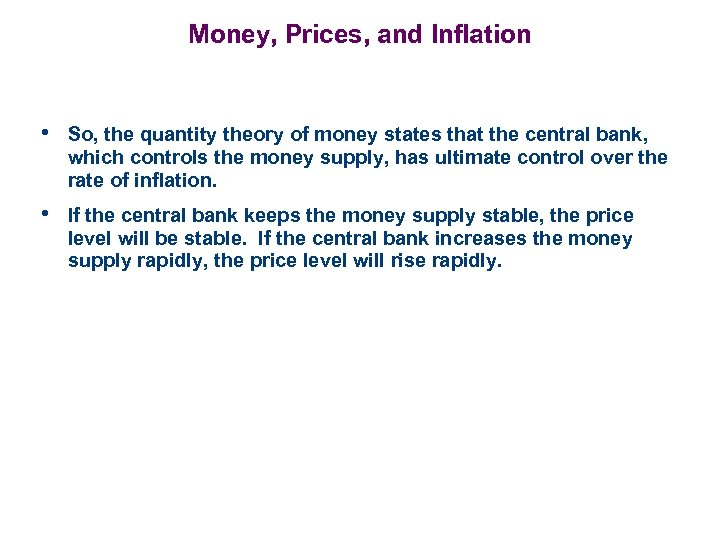Money, Prices, and Inflation • So, the quantity theory of money states that the central bank, which controls the money supply, has ultimate control over the rate of inflation. • If the central bank keeps the money supply stable, the price level will be stable. If the central bank increases the money supply rapidly, the price level will rise rapidly.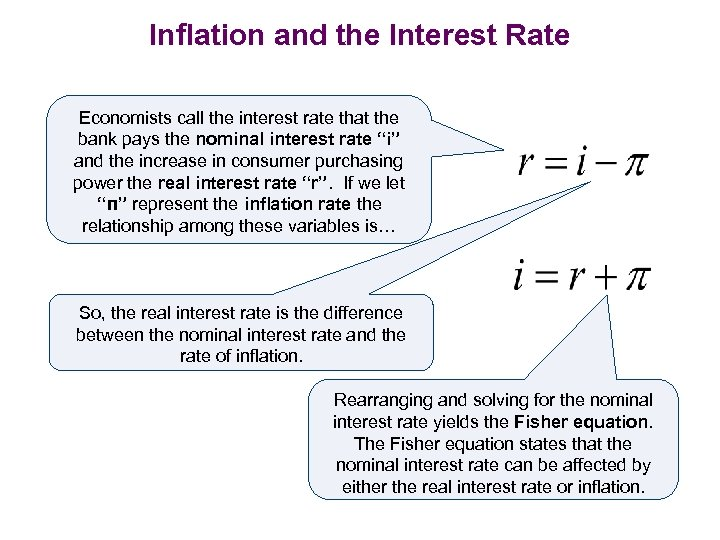Inflation and the Interest Rate Economists call the interest rate that the bank pays the nominal interest rate “i” and the increase in consumer purchasing power the real interest rate “r”. If we let “π” represent the inflation rate the relationship among these variables is… So, the real interest rate is the difference between the nominal interest rate and the rate of inflation. Rearranging and solving for the nominal interest rate yields the Fisher equation. The Fisher equation states that the nominal interest rate can be affected by either the real interest rate or inflation.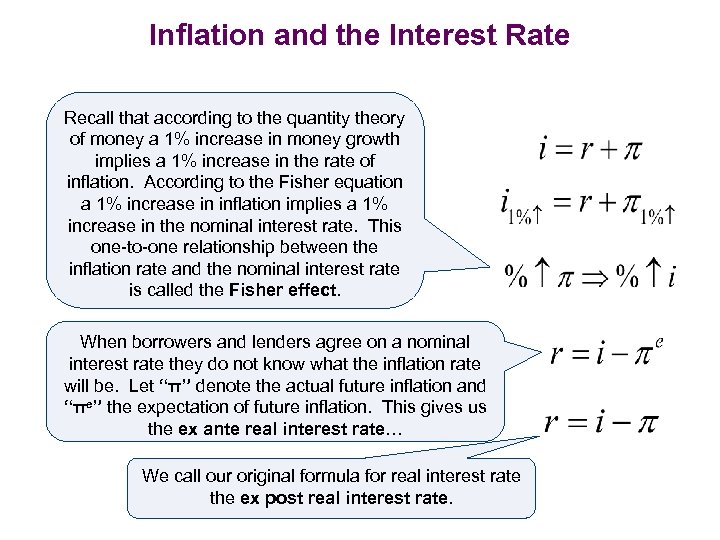Inflation and the Interest Rate Recall that according to the quantity theory of money a 1% increase in money growth implies a 1% increase in the rate of inflation. According to the Fisher equation a 1% increase in inflation implies a 1% increase in the nominal interest rate. This one-to-one relationship between the inflation rate and the nominal interest rate is called the Fisher effect. When borrowers and lenders agree on a nominal interest rate they do not know what the inflation rate will be. Let “π” denote the actual future inflation and “πe” the expectation of future inflation. This gives us the ex ante real interest rate… We call our original formula for real interest rate the ex post real interest rate.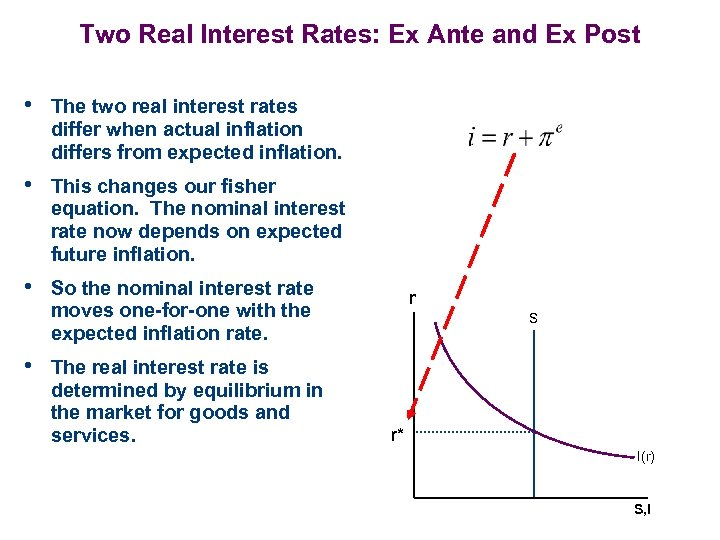Two Real Interest Rates: Ex Ante and Ex Post • The two real interest rates differ when actual inflation differs from expected inflation. • This changes our fisher equation. The nominal interest rate now depends on expected future inflation. • So the nominal interest rate r moves one-for-one with the expected inflation rate. S • The real interest rate is determined by equilibrium in the market for goods and services. r* I(r) S, I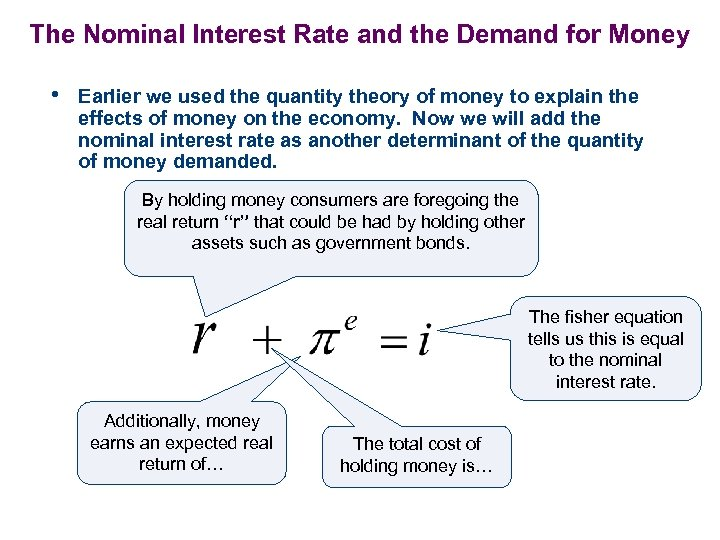The Nominal Interest Rate and the Demand for Money • Earlier we used the quantity theory of money to explain the effects of money on the economy. Now we will add the nominal interest rate as another determinant of the quantity of money demanded. By holding money consumers are foregoing the real return “r” that could be had by holding other assets such as government bonds. The fisher equation tells us this is equal to the nominal interest rate. Additionally, money earns an expected real return of… The total cost of holding money is…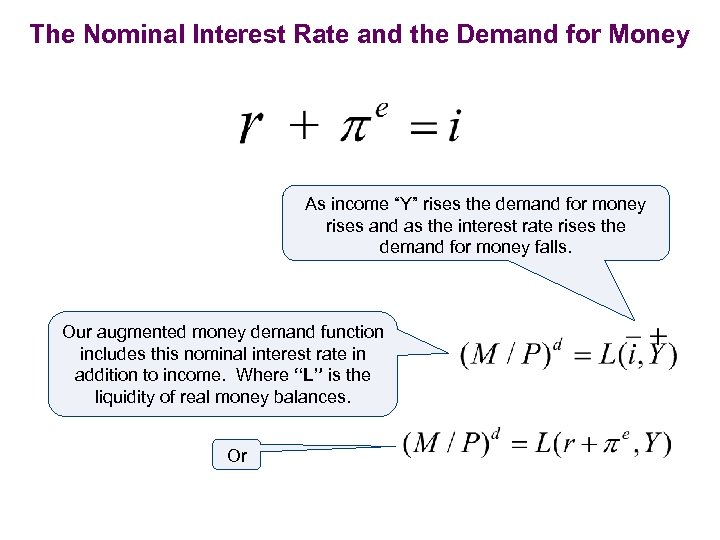The Nominal Interest Rate and the Demand for Money As income “Y” rises the demand for money rises and as the interest rate rises the demand for money falls. Our augmented money demand function includes this nominal interest rate in addition to income. Where “L” is the liquidity of real money balances. Or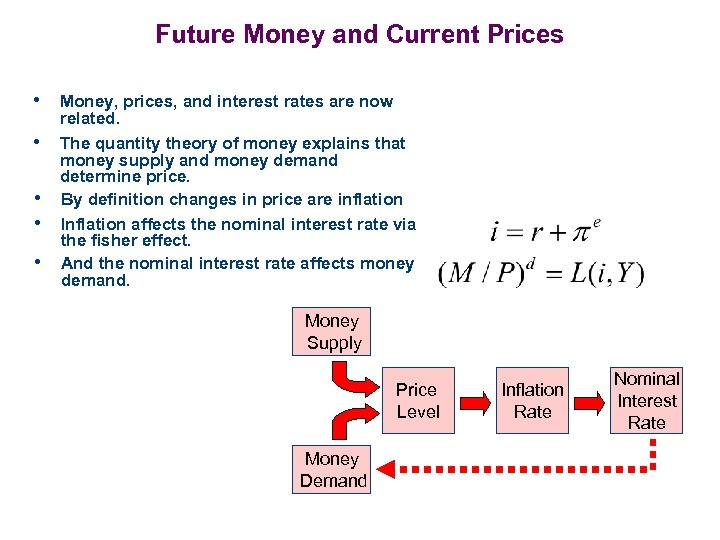Future Money and Current Prices • Money, prices, and interest rates are now • • related. The quantity theory of money explains that money supply and money demand determine price. By definition changes in price are inflation Inflation affects the nominal interest rate via the fisher effect. And the nominal interest rate affects money demand. Money Supply Price Level Money Demand Inflation Rate Nominal Interest Rate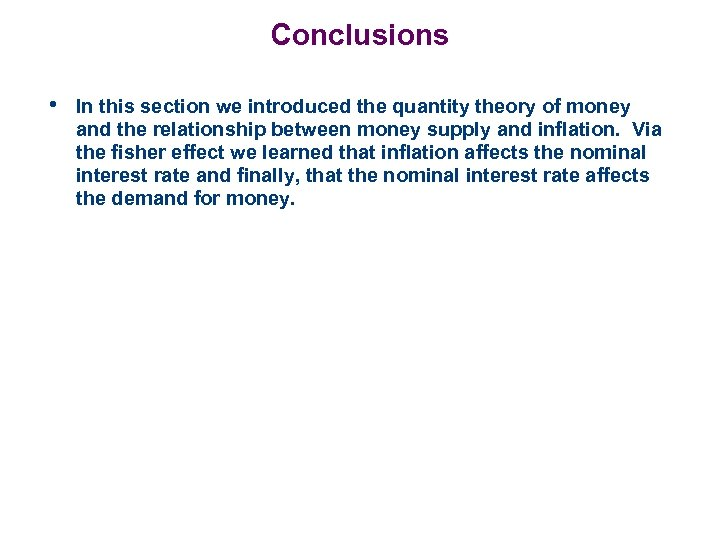Conclusions • In this section we introduced the quantity theory of money and the relationship between money supply and inflation. Via the fisher effect we learned that inflation affects the nominal interest rate and finally, that the nominal interest rate affects the demand for money.